American Journal of Theoretical and Applied Statistics
Volume 5, Issue 3, May 2016, Pages: 146-153

On Consistency of Tests for Stationarity in Autoregressive and Moving Average Models of Different Orders

Akeyede Imam1, *, Danjuma Habiba2, Bature Tajudeen Atanda3

1Department of Mathematics, Federal University, Lafia, Nigeria

2Department of Statistics, Federal Polytechnic Bali, Taraba State, Nigeria

3Department of Mathematics, Statistics Kwara State Polytechnic Ilorin, Kwara State, Nigeria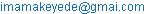(A. Imam)

*Corresponding author

Akeyede Imam, Danjuma Habiba, Bature Tajudeen Atanda. On Consistency of Tests for Stationarity in Autoregressive and Moving Average Models of Different Orders. American Journal of Theoretical and Applied Statistics. Vol. 5, No. 3, 2016, pp. 146-153. doi: 10.11648/j.ajtas.20160503.20

Received: April 26, 2016; Accepted: May 11, 2016; Published: May 25, 2016

Abstract: The most important assumptions about econometrics and time series data is stationarity, This study therefore suggests that, in trying to decide by classical methods whether economic data are stationary or not, it would be useful to perform tests of the null hypothesis of stationarity as well as tests of the null hypothesis of a unit root. The study compared power and type I error of Augmented Dickey-Fuller (ADF), Kwiatkowski, Phillips, Schmidt and Shin (KPSS) and Phillips and Perron (PP) to test the null hypothesis of stationarity against the alternative of a unit root at different order of autoregressive and moving average and various sample sizes. Simulation studies were conducted using R statistical package to investigate the performance of the tests of stationarity and unit root at sample size 20, 40, ..., 200 at first, second and third orders of autoregressive (AR), moving average (MA) and mixed autoregressive and moving average (ARMA) models. The relative performance of the tests was examined by their percentage of their powers and type I errors. The study concluded that PP is the best over all the conditions considered for the models, sample sizes and orders. However, in terms of type 1 error rate PP still is the best.

Contents

1. Introduction

The most important methods for dealing with econometrics and time series data, in the case of model fitting includes autoregressive (AR) models, moving average (MA) models, and mixed autoregressive moving average (ARMA) models. The basic assumption of these models is stationarity, that the data being fitted to them should be stationary.

Autoregressive and moving average models are mathematical models of the persistence, or autocorrelation, in a time series. The models are widely used in, econometrics, hydrology, engineering and other fields. There are several possible reasons for fitting AR, MA and ARMA models to data. Modeling can contribute to understanding the physical system by revealing something about the physical process that builds persistence into the series. The models can also be used to predict behavior of a time series or econometric data from past values. Such a prediction can be used as a baseline to evaluate the possible importance of other variables to the system. They are widely used for prediction of economic and industrial time series. Another use of AR, MA and ARMA models is simulation, in which synthetic series with the same persistence structure as an observed series can be generated. Simulations can be especially useful for established confidence intervals for statistics and estimated econometrics quantities.

These studies suggest that, in trying to decide by classical methods whether economic data are stationary or integrated, it would be useful to perform tests of the null hypothesis of stationarity as well as tests of the null hypothesis of a unit root. This paper provides a straight forward test of the null hypothesis of stationarity against the alternative of a unit root at different order of autoregressive and moving average and various sample sizes. There have been surprisingly few previous attempts to test the null hypothesis of stationarity.  Consider a test statistic which is essentially the F statistic for ‘superfluous’ deterministic trend variables; this statistic should be close to zero under the stationary null but not under the alternative of a unit root.  considers the Dickey-Fuller test statistics, but estimates both trend-stationary and difference-stationary models and then uses the bootstrap to evaluate the distribution of these statistics.

The outcomes of this research will assist a researcher to understand which of the tests of stationarity can be used for a particular order of autoregressive and moving average models and at what sample size is reliable.

1.1. Autoregressive Processes

An autoregressive model is simply a linear regression of the current value of the series against one or more prior values of the series. The value of (p) is called the order of the AR model. AR models can be analyzed with one of various methods, including standard linear least squares techniques.

Assume that a current value of the series is linearly dependent upon its previous value, with some error. Then we could have the linear relationship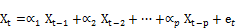(1)

Where,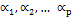are autoregressive parameters and et is a white noise process with zero mean and variance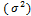.

Autoregressive processes are as their name suggests regressions on themselves. Specifically, a pth-order autoregressive process {Xt} satisfies the equation 1. The current value of the series Yt is a linear combination of the p most recent past values of itself plus an "innovation" term et that incorporates everything new in the series at time t that is not explained by the past values. Thus, for every t, we assume that et is independent of Xt1, Xt2, Xt3,....  carried out the original work on autoregressive processes.

1.2. Moving Average Model

The general moving average model can be given as follows;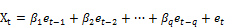(2)

We call such a series a moving average of order q and generally represented by MA(q). The terminology moving average arises from the fact that Xt is obtained by applying the weight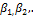..,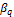to the variable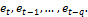Moving average model was first considered by  and later by .

1.3. Autoregressive Moving Average Model (ARMA)

In some application, the autoregressive or moving average model discussed in previous sections becomes cumbersome because we may need a higher order model with many parameters to adequately describe the dynamic structure of the data. To overcome these, Autoregressive Moving Average Model (ARMA) models are introduced . Basically an ARMA model combines the ideas of autoregressive and moving average models into a compact form so that the number of parameter used is kept small. The concept of ARMA model is highly relevant in volatility modeling .

If we assume that the series is partly autoregressive and partly moving average, we obtained a quite general time series model (ARMA).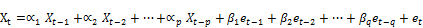(3)

We say that Xt, is an autoregressive and moving average of order p and q respectively. Symbolically, the model is represented by ARMA(p, q).

1.4. Stationarity

In Statistics, a stationary process is a stochastic process whose joint probability distribution does not change when shifted in time. Consequently, parameters such as the mean and variance, if they are present, also do not change over time.

The most important assumption made about time series data is that of stationarity. The basic idea of stationarity is that the probability laws that govern the behavior of the process do not change over time. In indeed, the process is statistically equilibrium. Specifically, a process {Yt} is said to be strictly stationary if the joint distribution of Yt is the same as that of Ytk for all t and k;

t = 1, 2, … k. In other words, the Y’s are (marginally) identically distributed [8,9]. It then follows that E(Yt) = E(Ytk) for all t and k so that the mean function is constant for all time. Additionally, Var(Yt) = Var(Ytk) for all t and k so that the variance is also constant over time. Also, the basic assumption of stationary time series is white noise, i.e the error term of the model must be normally distributed with mean zero and variance σ2.

Based on these, the parameter of autoregressive model fixed for our simulation were derived as p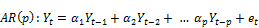(4)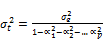(5)

The first order autoregressive is stationary when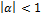obtained from the characteristic equation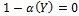.

For the second order autoregressive we introduced the autoregressive characteristic polynomial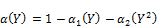and the corresponding AR characteristic equation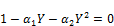(6)

It may be shown that, subject to the condition that et is independent of Yt1, Yt2, Yt3,..., a stationary solution to Equation (4) exists if and only if the roots of the AR characteristic equation exceed 1 in absolute value (modulus). We sometimes say that the roots should lie outside the unit circle in the complex plane. This statement will generalize to the pth-order case without change

In the second-order case, the roots of the quadratic characteristic equation (6) are easily found to be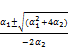(7)

The AR(2) is stationary if the absolute value of (7) exceed 1 (Jonathan and Kung-Sik, 2008 and Akeyede et al, 2015)

2. Methodology

Simulation studies were conducted to investigate the performance of Tests of stationarity in different order of autoregressive and moving average. Several data sets were simulated following assumption of stationarity earlier mentioned at different sample sizes and orders of autoregressive (AR) models, moving average (MA) models, and mixed autoregressive moving average (ARMA) models. Effect of sample size, order of autoregressive and the stationarity of the two models were examined on the power of stationarity tests. The tests that were considered in this study are augmented Dickey–Fuller test (ADF), Kwiatkowski, Phillips, Schmidt and Shin (KPSS) and Philip Perron (PP) tests

To illustrate the important statistical issues associated with autoregressive unit root tests, consider the simple AR(1) model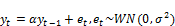H0: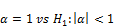The test statistic is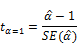Where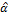is the least square estimate and SE(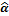) is usual standard error estimate The test is a one-sided left tail test. If yt is stationary (i.e.), then it can be shown by  that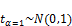2.1. Augmented Dickey–Fuller Test

In statistics and econometrics, an augmented Dickey–Fuller test (ADF) is a test for a unit root in a time series sample. It is an augmented version of the Dickey–Fuller test for a larger and more complicated set of time series models. The augmented Dickey–Fuller (ADF) statistic, used in the test, is a negative number. The more negative it is, the stronger the rejection of the hypothesis that there is a unit roots at some level of confidence.

2.2. Testing Procedure

The testing procedure for the ADF test is the same as for the Dickey–Fuller test but it is applied to the model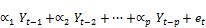(8)

By including lags of the order p the ADF formulation allows for higher-order autoregressive processes. This means that the lag length p has to be determined when applying the test. One possible approach is to test down from high orders and examine the t-values on coefficients. An alternative approach is to examine information criteria such as the Akaike information criterion, Bayesian information criterion or the Hannan–Quinn information criterion.

The unit root test is then carried out under the null hypothesis α = 0 against the alternative hypothesis of α <0 Once a value for the test statistic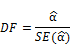is computed it can be compared to the relevant critical value for the Dickey–Fuller Test. If the test statistic is less (this test is non symmetrical so we do not consider an absolute value) than the (larger negative) critical value, then the null hypothesis of α = 0 is rejected and no unit root is present. 

2.3. Kwiatkowski, Phillips, Schmidt and Shin (KPSS)

 proposed an LM test for testing trend and/or level stationarity (the KPSS test). That is, now the null hypothesis is a stationary process. Taking the null hypothesis as a stationary process and the unit root as an alternative is in accordance with a conservative testing strategy. Hence, if we then reject the null hypothesis, we can be pretty confident that the series indeed has a unit root. Therefore, if the results of the tests above indicate a unit root but the result of the KPSS test indicates a stationary process, one should be cautious and opt for the latter result

Null hypothesis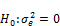Under the null hypothesis of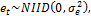the test statistic is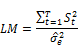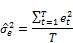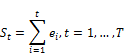where et are the residuals from the regression of yt on a constant and a time trend

2.4. Phillips Perron Test

The Phillips perron test (named after Peter C.B. Phillips and perron) is a unit root test. That is, it is used in time series analysis to test the null hypothesis that a time series is integrated of order 1. It builds on the Dickey–Fuller test of the null hypothesis in where the first difference operator. Like augmented Dickey–Fuller test, the Phillips Perron test addresses the issue that the process generating data for might have a higher order of autocorrelation than is admitted in the test equation making endogenous and thus invalidating the Dickey–Fuller test. Whilst the augmented Dickey–Fuller test addresses this issue by introducing lags of as regression in the test equation, the Phillips Perron test makes a non –parametric correlation to the t- test statistics. The test is robust with request to unspecified autocorrelation and heteroscedasticity in the disturbance process of the test equation.  report that the Phillips Perron test performs worse in finite samples than the augmented Dickey–Fuller test. And the advantage of the Phillips Perron test is that it is non–parametric, i.e it does not require to select the level of serial correlation as in ADF. It rather takes the same estimation scheme as in DF test, but corrects the statistic to conduct for autocorrelations and heteroscedasticity [14,15].

2.5. Methods of Simulations and Analyses

A set of replication of data sets are generated from first, second and third orders from autoregressive, moving average, and autoregressive moving average stated in 1, 2 and 3.

Where,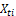are present responses simulated from random samples of normal distribution and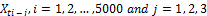are past responses of first and second order respectively.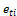is a random error which is known as white noise which is also distributed from normal distributions as follows: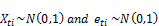For the simulation study, the choice of parameters considered by  was considered to ensure stationarity of the data generated for AR, MA and ARMA models. These are fixed as fixed as 0.1, 0.2, 0.3 and 0.5. The sample sizes simulated from each models are;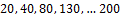. At a particular choice of sample size, the simulation study was performed 5000 times for different models.

Different methods of stationarity/ unit root tests were used to analyze each set of data simulated. The test statistic are ADF, KPSS and PP, the comparison were made by counting the number of times each test accepted the stationarity of the data simulated in 5000 replication. The percentages of their acceptance were recorded in table 1-6 for each case of the models, orders of autoregressive and moving average and sample sizes. The test with higher acceptance was considered as the best.

The variation in comparison of three stationarity/unit root tests will provide an indication of the sensitivity of the methods. Thus, the best method(s) were recommended for the research with various sample sizes, models and orders of autoregressive and moving average.

The outcomes of this research will assist a researcher to understand which of the tests of stationarity can be used for a particular order of autoregressive and moving average models and at what sample size is reliable.

2.6. Data Analyses

The results of the three methods of stationarity tests were presented in table 1 – 6 for the first, second and third orders of AR, MA and ARMA models. Data were simulated using R software package, to investigate the performances ADF, KPSS and PP on the simulated data at sample size 20, 40, 60, 80, 100, 120, 140, 160, 180 and 200. The relative performance of the tests were observed at order (p) = 1, 2 and 3 for data generated from different forms of autoregressive (AR) moving average (MA) and autoregressive moving average models (ARMA). The assumptions of stationarity were observed for cases of data generated. The relative performance of the stationarity tests was examined. The experiments were repeated 5000 times for the three Statistics and each sample size. The percentages of their acceptance were recorded in table 1-6

Table 1. Percentage of correctly Identifying Stationarity in Stationary Autoregressive Models (AR).

 ADF KPSS PP Order 1 2 3 1 2 3 1 2 3 Sample Size 20 99.98 56.76 95.94 92.10 49.30 76.92 100 100 100 40 99.98 57.74 96.16 92.46 49.98 76.20 100 100 100 60 99.98 57.94 95.92 92.86 51.40 76.86 100 100 100 80 99.98 57.04 95.90 92.96 50.76 75.44 100 100 100 100 99.98 57.34 95.90 92.24 50.66 76.04 100 100 100 120 99.98 58.72 96.04 92.04 49.02 76.18 100 100 100 140 99.98 58.32 95.76 92.42 50.48 76.96 100 100 100 160 99.98 57.88 95.12 92.70 51,64 76.30 100 100 100 180 99.94 57.56 95.98 92.32 50.98 76.40 100 100 100 200 99.98 57.20 95.76 92.46 49.60 76.10 100 100 100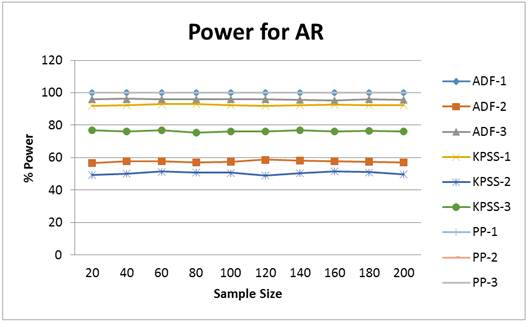Fig. 1. Power of Stationarity Test in AR models of Different Orders.

Table 2. Percentage of correctly Identifying Stationarity in Stationary Moving Average Models (MA).

 ADF KPSS PP Order 1 2 3 1 2 3 1 2 3 Sample Size 20 100.00 99.72 99.96 93.50 91.08 90.84 100 100 100 40 100.00 99.84 99.96 94.30 91.34 91.10 100 100 100 60 100.00 99.78 99.94 93.66 90.44 91.46 100 100 100 80 100.00 99.84 99.90 93.98 90.48 91.06 100 100 100 100 100.00 99.90 99.98 94.18 91.08 91.52 100 100 100 120 100.00 99.88 99.98 93.98 91.32 91.46 100 100 100 140 100.00 99.78 99.96 93.62 90.78 91.06 100 100 100 160 100.00 99.78 99.96 94.24 91.48 92.00 100 100 100 180 99.98 99.88 99.96 94.54 90.58 91.04 100 100 100 200 100.00 99.60 99.96 93.98 91.36 91.14 100 100 100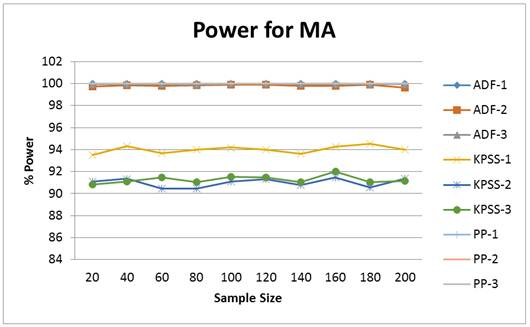Fig. 2. Power of Stationarity Test in MA models of Different Orders.

Table 3. Percentage of correctly Identifying Stationarity in Stationary Autoregressive and Moving Average (ARMA) Models.

 ADF KPSS PP Order 1 2 3 1 2 3 1 2 3 Sample Size 20 99.92 97.68 75.64 92.52 83.52 61.08 100 100 99.92 40 99.96 97.50 77.10 92.00 82.58 61.20 100 100 99.92 60 99.98 97.56 76.12 92.54 82.16 61.58 100 100 92.88 80 99.96 97.70 76.82 92.78 82.18 61.72 100 100 99.90 100 99.98 97.62 76.54 91.98 83.10 61.28 100 100 99.84 120 99.94 97.40 77.44 92.70 83.58 60.74 100 100 99.84 140 100.00 98.00 75.18 92.86 82.04 62.16 100 100 99.92 160 99.98 97.62 76.02 92.92 82.34 60.84 100 100 99.88 180 100.00 97.48 76.42 92.66 81.70 62.60 100 100 99.88 200 99.96 97.80 76.60 91.78 82.30 60.80 100 100 99.90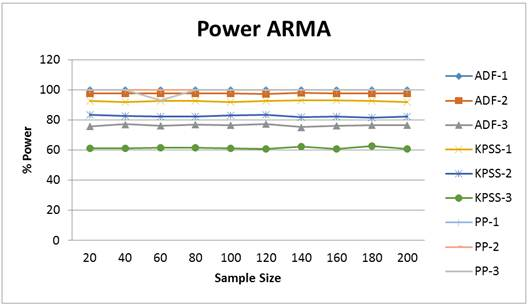Fig. 3. Power of Stationarity Test in ARMA models of Different Orders.

Table 4. Percentage of wrongly Identifying Stationarity in Non Stationary Autoregressive Models.

 ADF KPSS PP Order 1 2 3 1 2 3 1 2 3 Sample Size 20 4.44 5.08 5.04 3.38 1.40 2.36 1.84 0.00 0.04 40 4.38 5.52 4.90 3.22 1.74 2.26 2.14 0.00 0.14 60 4.60 5.28 4.48 3.10 1.68 2.16 2.10 0.00 0.00 80 5.12 5.24 4.70 3.40 1.54 2.42 1.90 0.02 0.08 100 4.72 4.96 4.68 3.36 1.48 2.34 1.80 0.00 0.08 120 4.68 5.18 4.34 2.78 1.54 4.96 1.88 0.02 0.08 140 5.04 5.42 4.78 3.26 1.44 2.62 1.78 0.00 0.04 160 4.70 5.44 5.02 3.48 1.82 2.46 1.84 0.00 0.16 180 5.20 4.44 5.16 3.32 1.40 2.34 2.04 0.00 0.10 200 4.92 4.98 4.92 2.36 1.70 2.12 1.90 0.02 0.10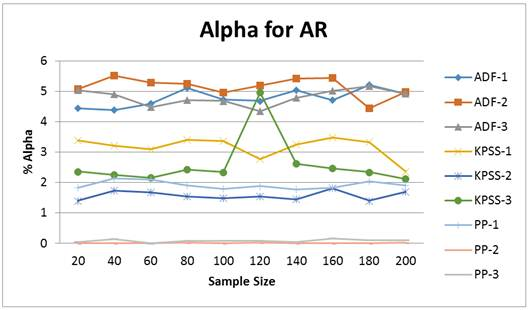Fig. 4. Type I error of Stationarity Test in AR models of Different Orders.

Table 5. Percentage of wrongly Identifying Stationarity in Non Stationary Moving Average Models (MA).

 ADF KPSS PP Order 1 2 3 1 2 3 1 2 3 Sample Size 20 4.86 6.60 4.54 3.26 3.18 3.04 2.72 1.18 1.56 40 4.66 6.60 4.82 3.14 3.18 3.18 2.78 1.36 1.62 60 4.92 6.42 4.38 3.00 3.98 3.12 3.14 1.32 1.38 80 4.72 6.08 4.56 3.34 2.84 2.78 2.90 1.18 1.60 100 4.36 5.88 4.08 3.28 3.10 2.94 2.68 1.62 1.30 120 4.60 5.92 4.42 3.02 2.92 2.88 2.74 1.46 1.16 140 4.80 6.18 4.90 3.38 3.32 3.40 3.14 1.28 1.40 160 4.42 6.10 4.48 2.94 3.18 3.06 2.98 1.20 1.40 180 4.92 5.96 4.10 3.36 2.78 3.08 3.36 1.16 1.44 200 4.60 6.28 4.92 3.56 2.80 2.96 3.24 1.36 1.46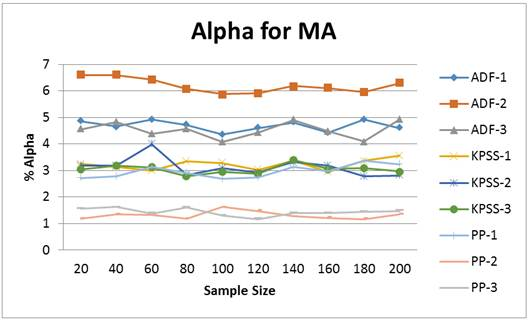Fig. 5. Type I Error of Stationarity Test in MA models of Different Orders.

Table 6. Percentage of wrongly Identifying Stationarity in Non Stationary Autoregressive and Moving Average (ARMA) Models.

 ADF KPSS PP Order 1 2 3 1 2 3 1 2 3 Sample Size 20 4.34 7.66 4.74 3.06 2.82 1.96 1.98 0.30 0.02 40 5.34 8.90 4.00 3.40 2.36 1.56 1.88 0.14 0.02 60 5.04 7.94 4.34 3.36 2.40 2.04 2.14 0.34 0.02 80 4.70 8.72 4.48 2.94 2.50 2.06 1.72 0.24 0.00 100 4.82 8.34 4.90 3.00 2.74 1.94 1.82 0.14 0.02 120 4.86 7.54 4.48 3.52 2.74 2.08 1.86 0.24 0.04 140 4.42 7.16 4.94 2.92 2.64 1.92 1.74 0.06 0.00 160 4.44 8.08 4.68 3.24 3.06 1.78 1.48 0.28 0.00 180 4.68 8.48 4.90 3.06 2.86 2.28 1.80 0.24 0.00 200 4.80 8.82 4.64 3.36 2.54 2.18 1.82 0.34 0.00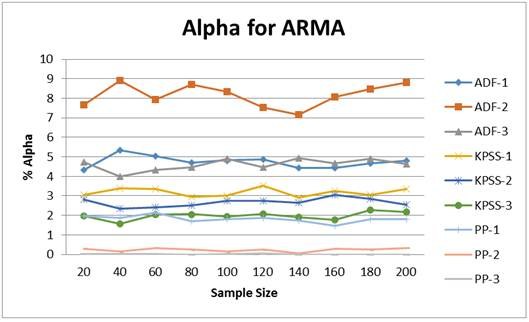Fig. 6. Type I Error of Stationarity Test in AR MA models of Different Orders.

3. Conclusion

Table 1 presents the result of the power for all the test procedure when the underlying time series model is stationary AR. All the procedures produced a reasonably high power over all the sample sizes and order considered except at order 2 where ADF (Augmented Dickey Fuller) and KPSS produced extremely low power compared to PP. Under this condition, Philip-Peron (PP) has the highest power over all the sample sizes and AR orders considered. Table 2 presents similar analysis on stationary MA, the power of the tests are extremely high over all the sample sizes and orders considered. Similar conclusion as in AR was also observed here. Table 3 presents the power of the mixed model (Stationary ARMA), all the test procedures produced high power over all the sample sizes at order 1 but ADF and KPSS produced low power over all the sample size at order 2 & 3.

Table 4 presents the empirical percentage type 1 error rate of the test over the sample sizes and order for Non-stationary AR models, the main focus here is to compare the empirical type 1 error rate with the nominal 5%. Out of all the test procedures only PP produced a reasonably closer estimate to the nominal 5% level with considerably improvement as the order increases. The worst of all the test procedure is ADF under this condition. Table 5 also presents the result under Non-stationary MA models, PP seem to produced the best estimate but not as in table 4 them followed by KPSS and ADF is the worst. Finally, table 6 presents ARMA results on empirical percentage type 1 error rate, PP test is still to be the best procedure under this condition but the performance seems to be poor at order 1.

Also, from Fig 1—6 have the same results as stated in table 1—6 above.

Generally speaking, in terms of validity of the test based on percentage power, PP is the best over all the conditions considered which are models, sample sizes and order. But in terms of usability of the test that is based on type 1 error rate PP still is the best.

Recommendation

The PP is better applied since it produced reasonably high power and closer estimate of type 1 error to the nominal value. The above conclusion implies that pp test correctly rejects and at the same time identifies when not to reject.

References

1. Park, J. Y. and B. Choi, (1988), A new approach to testing for a unit root, Working paper no. 88 23(Center for Analytical Economics, Cornell University, Ithaca, NY).
2. Rudebusch, G. D., (1990), Trends and random walks in macroeconomic time series: A reexamination, Working paper no. 105 (Economic Activity Section, Board of Governors of the Federal Reserve System, Washington, DC).
3. Yule, G. U. (1926). "Why do we sometimes get nonsense-correlations between time-series? — A study in sampling and the nature of time-series." Journal of the Royal Statistical Society, 89, 1, 1–63.
4. Tsay, R. S. (2008). Analysis of Financial Time Series, Second Edition, A John Wiley & Sons, Inc., Publication, pp 75-77.
5. Tsay, R. S. (2010). Analysis of Financial Time Series; 3rd Edition, Published by John Wiley & Sons, Inc., Hoboken, New Jersey. Published simultaneously in Canada
6. Box, G. E. P., Jenkins, G. M., and Reinsel, G. C. (1994). Time Series Analysis, Forecasting and Control, 2nd ed. New York: Prentice-Hall.
7. Jonathan D. C. and Kung-Sik C. (2008). Time Series Analysis with Applications in R Second Edition, Springer Science+Business Media, LLC.
8. Choi I (2006). \Nonstationary Panels. In TC Mills, K Patterson (eds.), Econometric Theory, volume 1 of Palgrave Handbook of Econometrics, chapter 13, pp. 511{539. Palgrave MacMillan, New York.
9. Akeyede, I., Adeleke, B. L. and Yahya, W. B., (2015), Forecast strength of some linear and nonlinear time series data, American Journal of Mathematics and Statistics, 5(4): 37-41.
10. Hamilton, J. D. (1994). Time Series Analysis. Princeton University Press, Princeton, NJ.
11. Dickey, D. A., Fuller, W. A (1981). Likelihood Ratio Statistics for Autoregressive Time Series with a Unit Root." Econometrica, 49(4), 1057-1072.
12. Choi, I., (1990), Most of the US economic time series do not have unit roots: Nelson and Plosser’s results reconsidered, Discussion paper (Department of Economics, Ohio State University, Columbus, OH).
13. Kwiatkowski, D., Phillips, P. C. B., Schmidt, P., Shin, Y., (1992). Testing the null hypothesis of stationarity against the alternative of a unit root: How sure are we that economic time series have a unit root? Journal of Econometrics 54, 159–178
14. Davidson and Mackinnon (2004). Report that the Phillips Perron test performs worse in finite samples than the augmented Dickey–Fuller test, Journal of Economic Dynamics and Control 12, 297-332
15. Phillips, P. C. B. and P. Perron. (1988), Testing for a unit root in time series regression, Biometrika 75, 335-346.

 Contents 1. 1.1. 1.2. 1.3. 1.4. 2. 2.1. 2.2. 2.3. 2.4. 2.5. 2.6. 3.
Article ToolsAbstractPDF(1271K)